Author: Oscar Cronquist Article last updated on February 21, 2023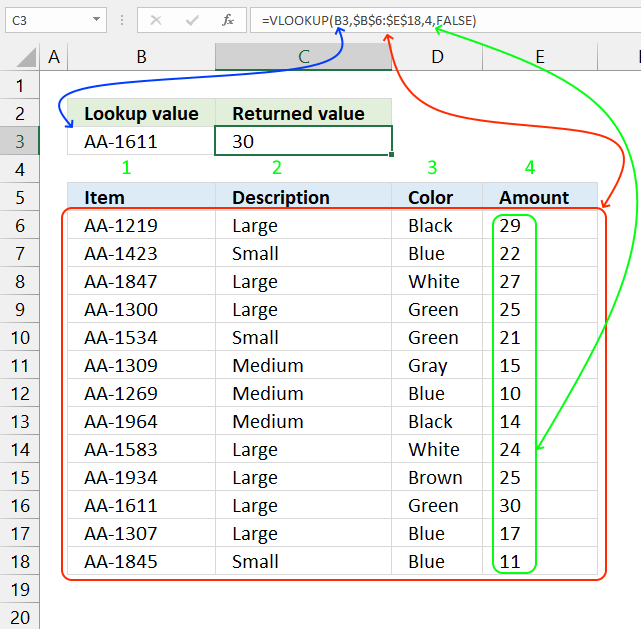The VLOOKUP function lets you search the leftmost column for a value and return another value on the same row in a column you specify.

Use the new XLOOKUP function which is an improved version of the VLOOKUP function available for Excel 365 users.

VLOOKUP stands for vertical lookup and your data (table_array) must be organized into records, a record on each row. To search records excel must look vertically in the data array. HLOOKUP stands for horizontal lookup but that is for another post.

## 1. VLOOKUP function syntax

VLOOKUP(lookup_value, table_array, col_index_num, [range_lookup])

## 2. VLOOKUP function arguments

 lookup_value Value you want to lookup. table_array The range you want to use, remember that the VLOOKUP function always looks in the leftmost column in your specified range. col_index_num The column number which contains the return value. [range_lookup] True or False (boolean value). True - approximate match, the leftmost column must be sorted ascending. False - Exact match.

## 3. VLOOKUP function formulaFormula in cell C3:

=VLOOKUP(B3, B6:E18, 4, FALSE)

The VLOOKUP function uses lookup value AA-1611 in cell B3 and searches Table1 (cell range B6:E18) in the leftmost column. A matching value is found on row 16.

The third argument is which column you want to fetch the corresponding value from. In this example, column 4 is entered and £30.00 is returned in cell C3.

The fourth and last argument lets you choose between approximate and exact match, in this case, FALSE meaning EXACT match.

The VLOOKUP function is designed to return only one value, however, there are workarounds to fetch all matching values.
5 easy ways to VLOOKUP and return multiple values
I recommend the FILTER function if you are an Excel 365 user.

## 4. VLOOKUP - approximate match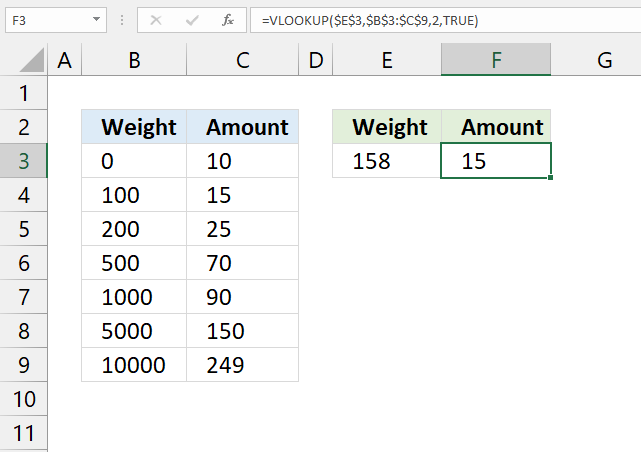The fourth argument [range_lookup] allows you to choose if you want an APPROXIMATE or EXACT match.

You want, in most cases, to use the EXACT match however the default value is an APPROXIMATE match. So make sure you know what you are doing.

True - Approximate match (default)
False - Exact match

This means that you get the approximate match if you don't specify the fourth argument at all.

VLOOKUP(lookup_value, table_array, col_index_num, [range_lookup])

The example above shows you the difference between EXACT and APPROXIMATE match. You must have your leftmost column sorted in an ascending order or you may get incorrect results.

The approximate match finds the largest value that is less than or equal to the lookup_value.

The approximate match is useful if you want to find which group or range your value belongs to. The example above shows that value 100 is the largest value that is less than or equal to 158, the corresponding value to 100 in column C is 15.

Formula in cell C3:

=VLOOKUP(E3, B3:C9, 2, TRUE)

Example weights and result amounts:

 Weight Amount 0 10 50 10 99 10 100 15 101 15 150 15 199 15 200 25 201 25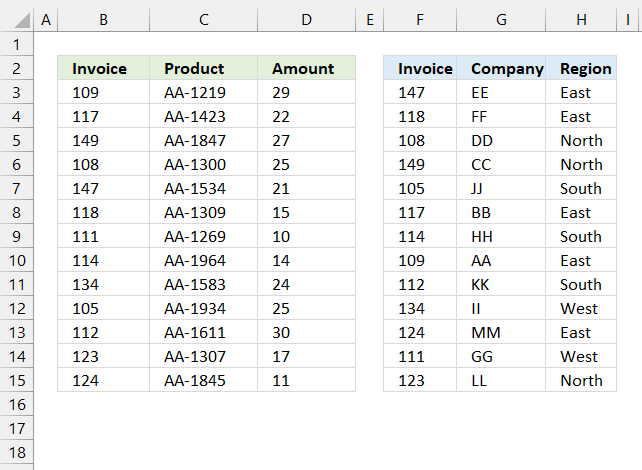A Pivot Table can't work with related tables (power pivots do) however the VLOOKUP function can quickly merge related tables so you then can analyze data in a Pivot Table.

These two tables are related meaning they share a column (invoice), you can use the following formula to merge these two data sets based on the invoice column, this will organize data and put corresponding data next to the appropriate invoice number.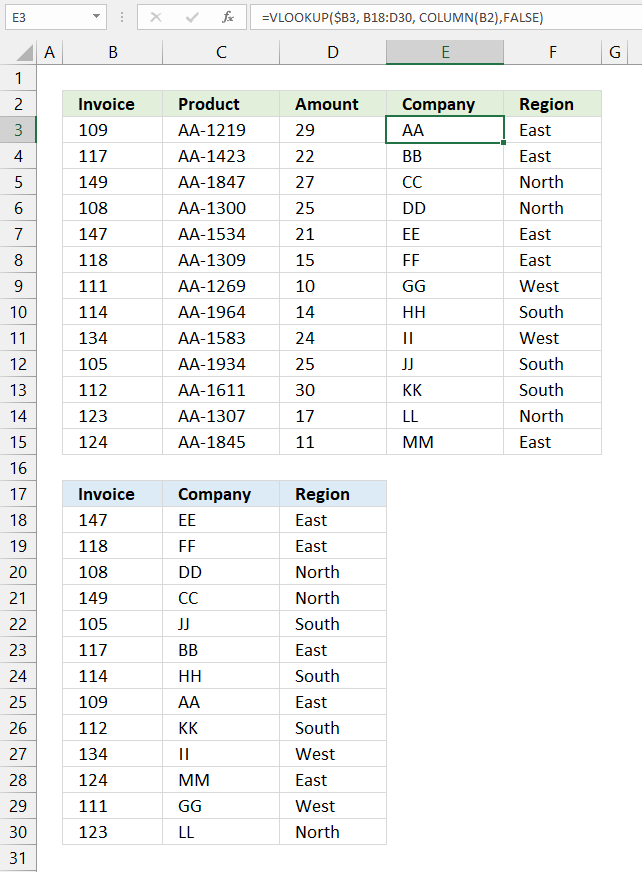The image above shows both data sets and the VLOOKUP function in cell E3.

Formula in cell E3:

=VLOOKUP(\$B3, B18:D30, COLUMN(B2), FALSE)

Copy formula in cell E3 and paste to E3:F15.

### Explaining formula in cell E3

#### Step 1 - Calculate column number to get values from

The COLUMN function returns a number representing the position of a column counting from left to right based on a cell reference.

We can use this to create a dynamic number that changes when we copy the formula and paste to adjacent cells.

The technique that makes this possible is a relative cell reference, in this case, cell reference B2.

COLUMN(B2) returns 2.

#### Step 2 - Change cell reference to a relative cell reference

We want to use values only from column B, use the dollar sign to lock the cell reference to column B.

This keeps the cell reference pointing to column B, however, the row part of the cell reference is relative meaning it will change when the formula is copied and pasted to other cells.

\$B3

#### Step 3 - Get value

VLOOKUP(\$B3, B18:D30, COLUMN(B2), FALSE)

becomes

VLOOKUP(109, {147, "EE", "East";118, "FF", "East";108, "DD", "North";149, "CC", "North";105, "JJ", "South";117, "BB", "East";114, "HH", "South";109, "AA", "East";112, "KK", "South";134, "II", "West";124, "MM", "East";111, "GG", "West";123, "LL", "North"}, {2}, FALSE)

and returns "AA"

## 6. How to use the VLOOKUP function with a cell reference specified in a cell?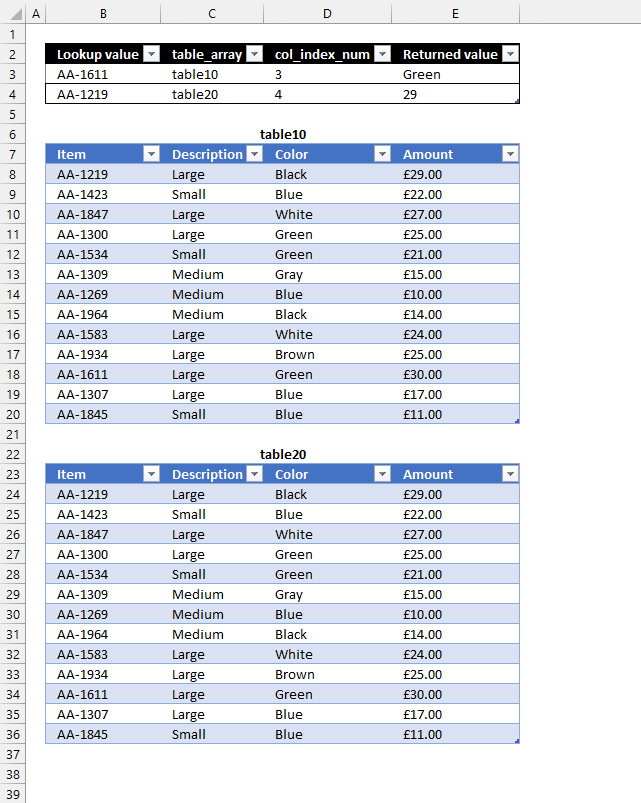This example shows you how to use the VLOOKUP function with multiple tables, it searches an Excel Table based on the specified table name in cell C3. The image above shows two Excel tables, table10 and table20.

Here is how it works, the lookup value is in B3, the Excel Table name is in C3, the column number in cell D3, and the formula is in cell E3.

The formula in cell E3 uses the values in B3, C3, and D3 to extract the appropriate value, this example shows how to use cell values instead of hardcoded values as arguments.

Formula in cell E3:

=VLOOKUP(B3,INDIRECT(C3),D3,FALSE)

### Explaining formula in cell E3

#### Step 1 - Convert text string to a cell reference

The INDIRECT function allows you to convert a string to a valid cell reference.

INDIRECT(C3)

becomes

INDIRECT("table10")

and returns table10.

#### Step 2 - Get value

VLOOKUP(B3, INDIRECT(C3), D3, FALSE)

becomes

VLOOKUP("AA-1611", table10, 3, FALSE)

and returns "Green" in cell E3.

The INDIRECT function lets you use a cell value as the table_array argument, this lets you quickly change the value in cell C3 and search multiple tables.

## 7. What if the lookup value is not in the leftmost column?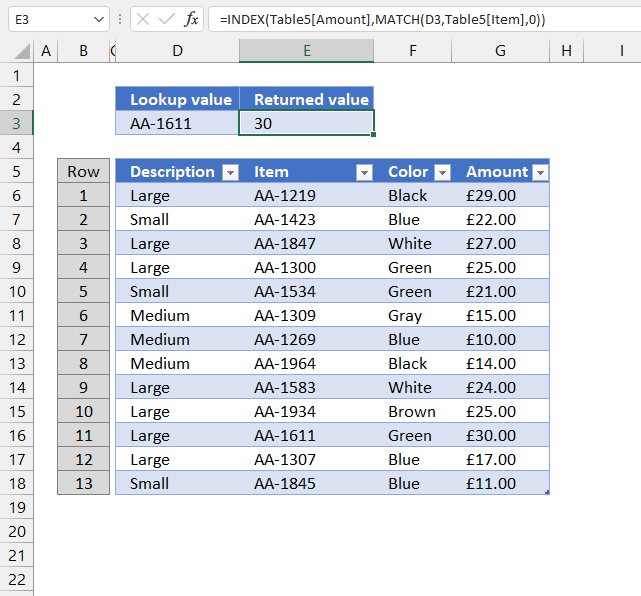The disadvantage with the VLOOKUP function is that it can only look for a value in the leftmost column. If you don't have your values in the leftmost column you have three options, rearrange your table, use the XLOOKUP function (Excel 365), or use another method demonstrated below.

The INDEX and MATCH function allows you to search any column in a table and return a value in any column on the same row. This formula is so versatile that I actually prefer INDEX + MATCH over the VLOOKUP function.

Formula in cell C3:

=INDEX(G6:G18, MATCH(D3, E6:E18, 0))

### Explaining formula in cell C3

#### Step 1 - Find the relative position of a lookup value

The MATCH function lets you look for a value in a column and return the relative position of the found value in the array.

MATCH(D3, E6:E18, 0)

becomes

MATCH("AA-1611", E6:E18, 0)

and returns 11. AA-1611 is found on row 11 in cell range E6:E18.

#### Step 2 - Get value

The INDEX function returns a value from a cell range, you specify which value based on a row and column number.

INDEX(array, [row_num], [column_num])

INDEX(G6:G18, MATCH(D3, E6:E18, 0))

becomes

INDEX(G6:G18, 11)

and returns 30 in cell C3.

## 8. How to handle VLOOKUP errorsThe IFERROR function allows you to return a value if the VLOOKUP function returns an error, in this case NOT FOUND!

## 9. How to use VLOOKUP in VBA?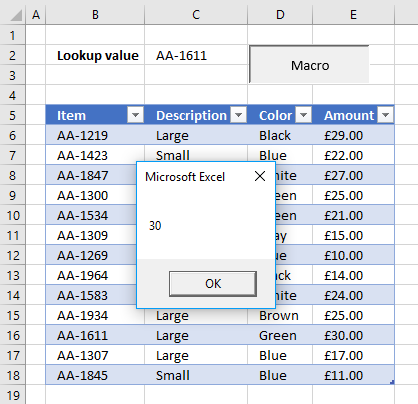The following VBA code demonstrates how to use VLOOKUP in a macro.

The macro searches B6:B18 using the value in cell C2 and displays the result in a message box.

```VBA code
Sub VLP()
MsgBox Application.WorksheetFunction.VLookup(Range("C2"), Range("B6:E18"), 4, False)
End Sub
```

## 11. VLOOKUP function - Partial match using wildcards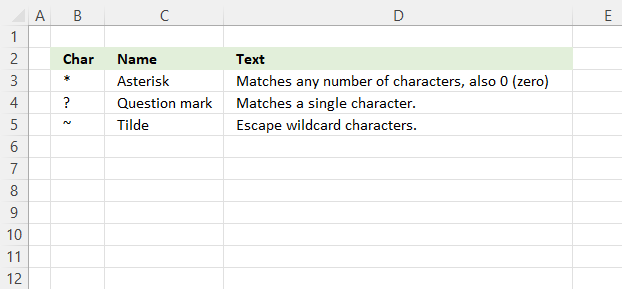The asterisk character lets you match any number of characters in sequence also zero. The question mark matches only a single character, however, this is very useful if you want to match a specific number of characters.

Use the tilde character to escape the asterisk or question mark in a VLOOKUP formula, in other words, it lets you use the actual asterisk or a question mark character in the lookup value.

### 11.1 VLOOKUP function - Partial match - begins with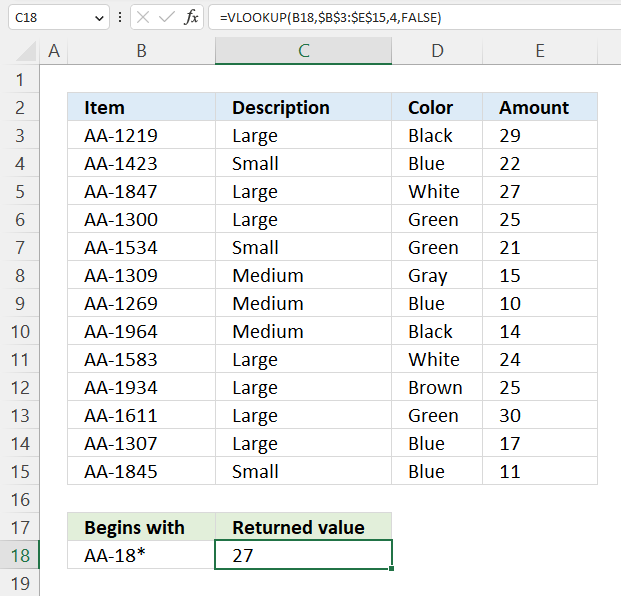This example demonstrates how to perform a partial match using the VLOOKUP function, specifically if a cell value begins with a given string.

The asterisk character lets you match 0 (zero) to any number of characters: Asterisk character

Formula in cell C18:

=VLOOKUP(B18,\$B\$3:\$E\$15,4,FALSE)

Cell B18 contains the condition, note that the asterisk is the last character. The VLOOKUP function returns a value in column E on the same row if a value in cell range B3:B15 matches the condition specified in cell B18.

### 11.2 VLOOKUP function - Partial match - ends with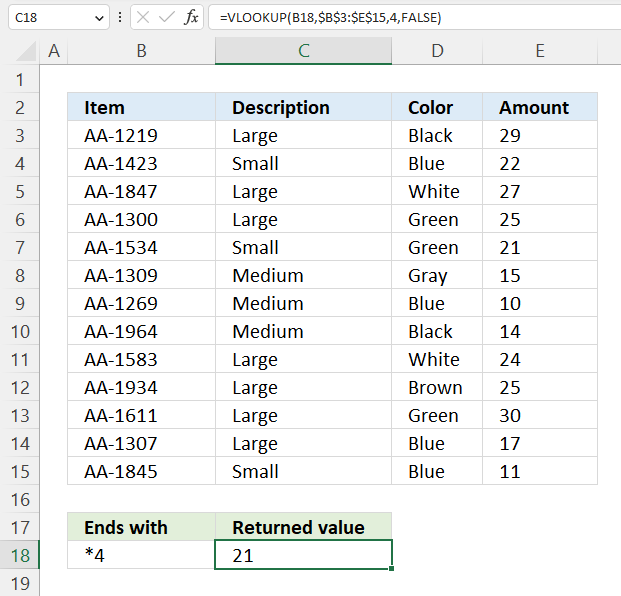The image above shows how to VLOOKUP using a partial match, the first value in cell range B3:B15 that ends with a 4 match. Cell B7 contains "AA-1534", the VLOOKUP formula returns the value on the same row from column E, in this case, value 21.

Formula in cell C18:

=VLOOKUP(B18,\$B\$3:\$E\$15,4,FALSE)

Note that the condition in cell B18 is "*4", the asterisk matches any number of characters also 0 (zero).

### 11.3 VLOOKUP function - Partial match - contains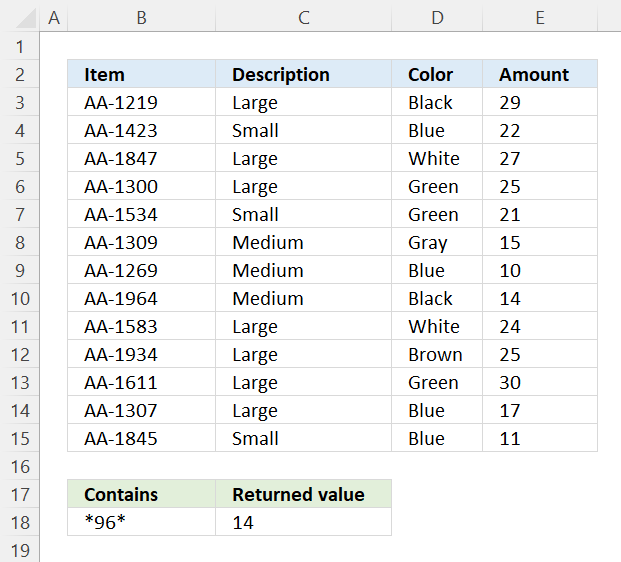The condition in cell B18 begins and ends with an asterisk, this technique lets you look for values that contain a given string, in this case, 96.

The first value in cell range B3:B15 that contains 96 is found in cell B10, the value on the same row in column E is 14 and is found in cell E10.

Formula in cell C18:

=VLOOKUP(B18,\$B\$3:\$E\$15,4,FALSE)

### 11.4 VLOOKUP function - Partial match - question mark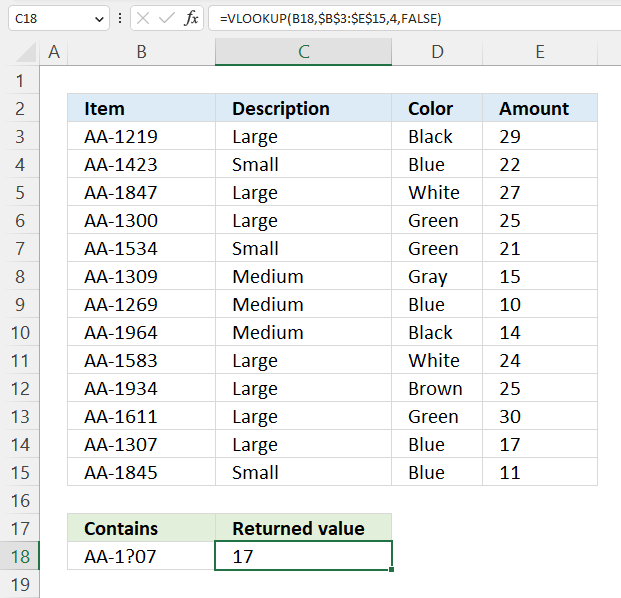Cell B18 contains the lookup value AA-1?07, and the question mark matches any single character.

Formula in cell C18:

=VLOOKUP(B18,\$B\$3:\$E\$15,4,FALSE)

The formula in cell C18 finds a match in cell B14 "AA-1307", the value on the same row in column E is 17.

### 11.5 VLOOKUP function - tilde character escapes wildcard characters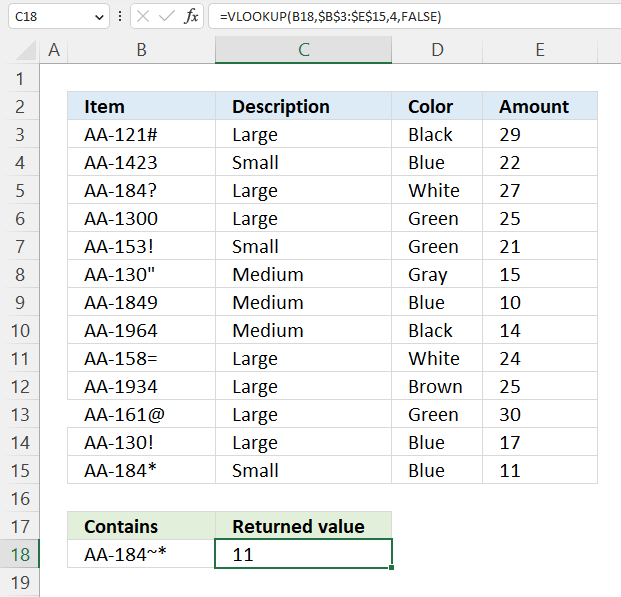The tilde character lets you escape wildcard characters in the lookup value, the image above demonstrates a tilde character in cell B18.

Formula in cell C18:

=VLOOKUP(B18,\$B\$3:\$E\$15,4,FALSE)

The VLOOKUP formula matches "AA-184~*" to "AA-184*" and not value "AA-184?".

## 12 VLOOKUP - Duplicate repeated values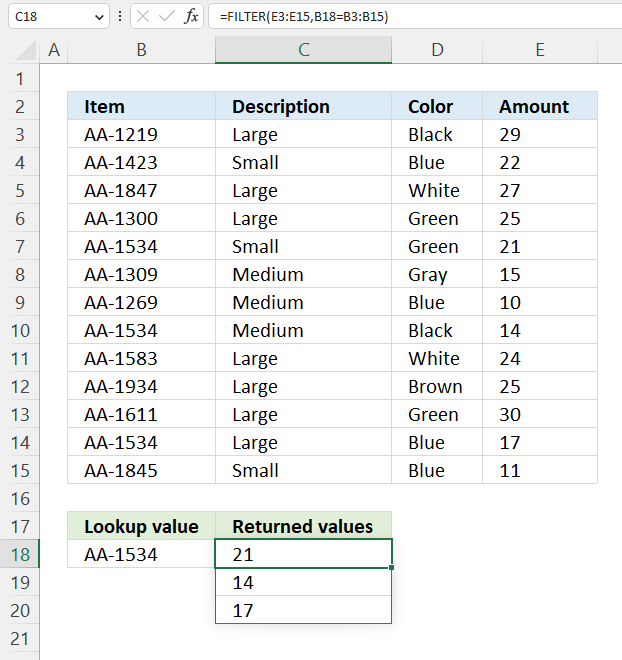The VLOOKUP can't return multiple values, however, the new Excel 365 FILTER function can. This example looks for "AA-1534" specified in cell B18, in cells B3:B15.

Formula in cell C18:

=FILTER(E3:E15,B18=B3:B15)

3 matches are found in cells B7, B10, and B14, values 21, 14, and 17 are returned to cell C18. This formula spills values automatically as far as needed below cell C18.

The FILTER function extracts values/rows based on a condition or criteria.

Function syntax: FILTER(array, include, [if_empty])

## 13. VLOOKUP and HLOOKUP together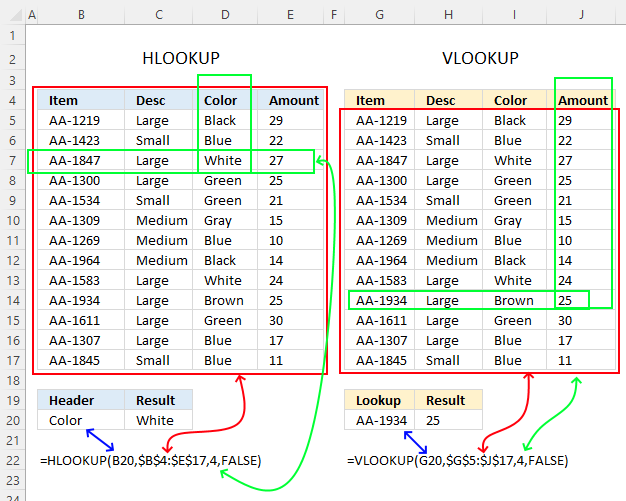I don't recommend using both the VLOOKUP and HLOOKUP functions in one formula to perform a horizontal and vertical lookup simultaneously (2D lookup), I don't think it's even possible combining the functions to get the desired result without making it overly complicated.

The image above demonstrates the differences between the two functions.

The HLOOKUP performs a horizontal lookup in the top row of a given cell range whereas the VLOOKUP function performs a vertical lookup in the left-most column.

VLOOKUP(lookup_value, table_array, col_index_num, [range_lookup])

HLOOKUP(lookup_value, table_array, row_index_num, [range_lookup])

Use the INDEX and MATCH functions to perform a 2d lookup (two-dimensional lookup), it is so much easier.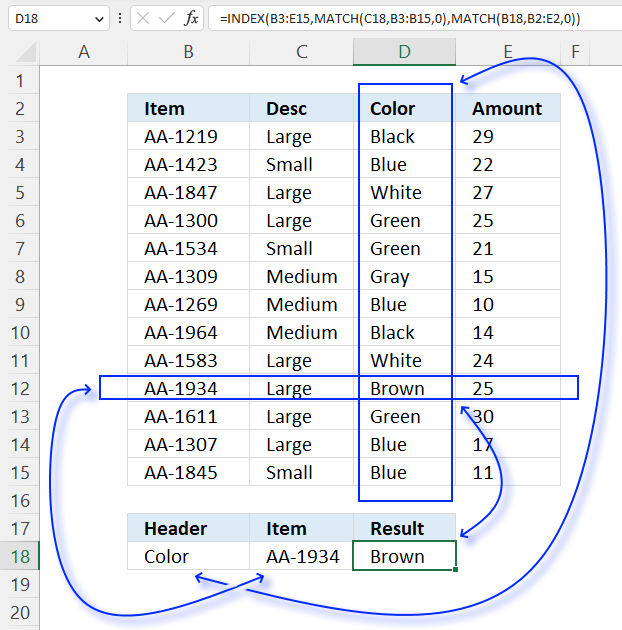The image above shows a formula that searches a table both horizontally and vertically, the formula in cell D18 uses two conditions to get the desired value.

The first condition specified in cell B18 makes a lookup horizontally across header names, the second condition specified in cell C18 performs a vertical lookup in column B. The value of the intersection of the matching column and row is returned to cell D18, in this example "Brown".

Formula in cell D18:

=INDEX(B3:E15, MATCH(C18, B3:B15, 0),MATCH(B18, B2:E2, 0))

You can find an explanation of the formula here: How to perform a two-dimensional lookup

## 14. VLOOKUP function can't find a value that is actually there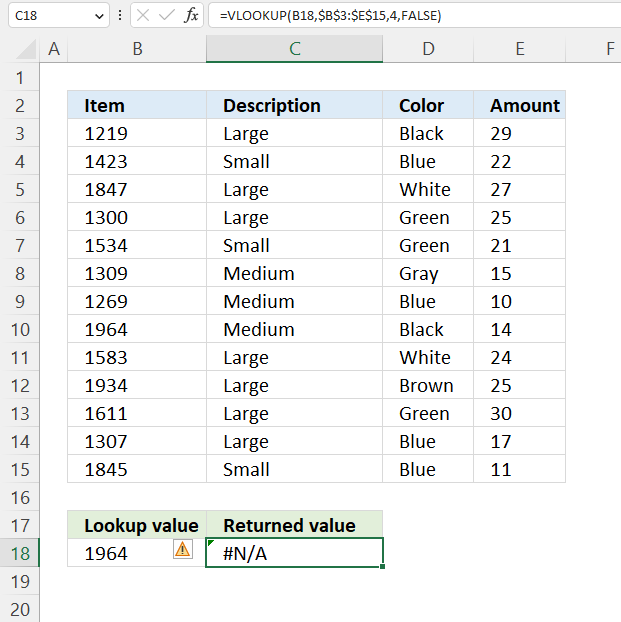Here are some reasons why the VLOOKUP function can't lookup a value that is actually there:

1. The values are formatted differently, for an example, if you try to lookup a number and the lookup column consists of text values. One way to identify numbers stored as text is if the number has a leading ' (apostrophe). This can happen if you import data into Excel from a database. The image above demonstrates this issue, cell B10 contains this value '1964, however, Excel "hides" the apostrophe automatically making it impossible to spot unless you select the cell and examine the contents of the formula bar.
2. The lookup value or the lookup column contains values with leading or trailing space characters. The image below shows how the TRIM function is able to remove leading and trailing spaces, you don't need to edit each value and remove the space characters.
3. The table must have the lookup-column in the left-most column of the specified cell range. There are a few possible ways to solve this:
1. Rearrange the columns manually.
2. Use another Excel function preferably the XLOOKUP function or the INDEX MATCH functions combined.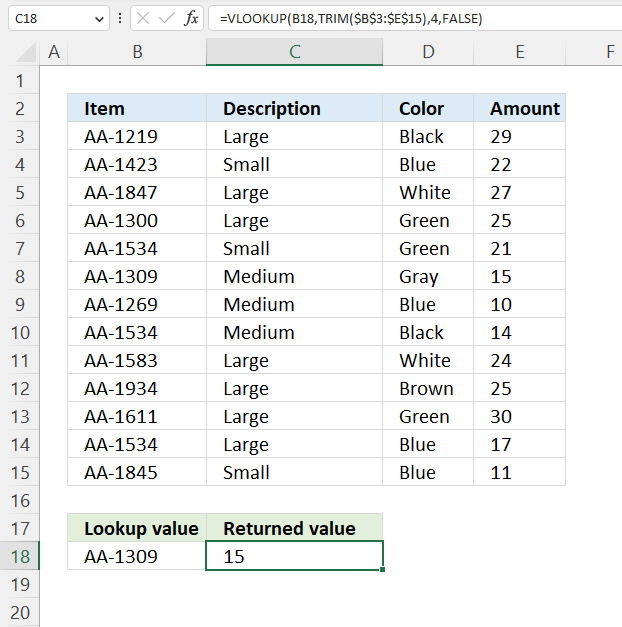The following formula removes leading and trailing space characters in cell range B3:E15. Cell B8 contains a trailing space, the TRIM function removes this space character so the VLOOKUP function can lookup the correct value.

Formula in cell C18:

=VLOOKUP(B18,TRIM(\$B\$3:\$E\$15),4,FALSE)

### Explaining formula

#### Step 1 - Delete leading and trailing spaces

The TRIM function deletes all blanks or space characters except single blanks between words in a cell value.

Function syntax: TRIM(text)

TRIM(\$B\$3:\$E\$15)

becomes

TRIM({"AA-1219","Large","Black",29; "AA-1423","Small","Blue",22; "AA-1847","Large","White",27; "AA-1300","Large","Green",25; "AA-1534","Small","Green",21; "AA-1309 ","Medium","Gray",15; "AA-1269","Medium","Blue",10; "AA-1534","Medium","Black",14; "AA-1583","Large","White",24; "AA-1934","Large","Brown",25; "AA-1611","Large","Green",30; "AA-1534","Large","Blue",17; "AA-1845","Small","Blue",11})

and returns

{"AA-1219","Large","Black","29"; "AA-1423","Small","Blue","22"; "AA-1847","Large","White","27"; "AA-1300","Large","Green","25"; "AA-1534","Small","Green","21"; "AA-1309","Medium","Gray","15"; "AA-1269","Medium","Blue","10"; "AA-1534","Medium","Black","14"; "AA-1583","Large","White","24"; "AA-1934","Large","Brown","25"; "AA-1611","Large","Green","30"; "AA-1534","Large","Blue","17"; "AA-1845","Small","Blue","11"}

#### Step 2 - Perform VLOOKUP

The VLOOKUP function lets you search the leftmost column for a value and return another value on the same row in a column you specify.

Function syntax: VLOOKUP(lookup_value, table_array, col_index_num, [range_lookup])

VLOOKUP(B18,TRIM(\$B\$3:\$E\$15),4,FALSE)

returns 15.

## 15. VLOOKUP alternatives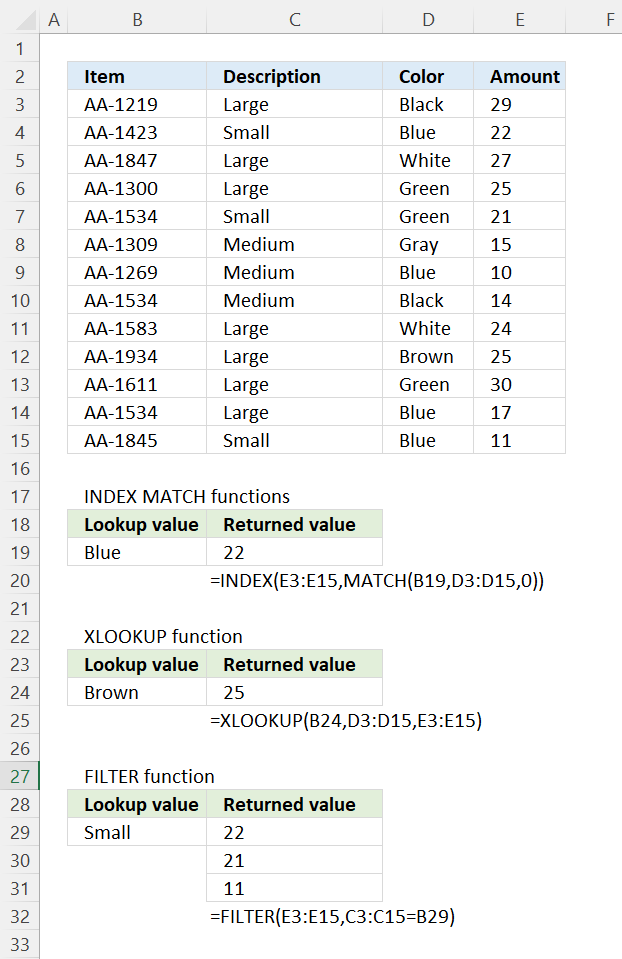Each of these alternatives has its own pros and cons, however, unfortunately, your Excel version determines which function you can use. The XLOOKUP and FILTER functions are only available in Excel 2019/Excel 365 and are more versatile than the VLOOKUP function.

In general, the INDEX and MATCH functions combined is a good alternative to VLOOKUP when you need more flexibility in your lookup formula, and they are available for almost all Excel versions.

The XLOOKUP function offers more flexibility and functionality than the VLOOKUP function. It allows you to search for a value in any column of a table and then return a value from a specified column in the same row, in other words, search for a value in a table even if the value you're looking for isn't in the leftmost column. Additionally, XLOOKUP allows you to specify multiple search criteria, which can make it easier to find the exact value you're looking for.

The FILTER function allows you to get data from any column or columns based on all matching values in the lookup column. Also, you can use multiple conditions on whatever column you like.

The LOOKUP function is a bad choice, it works best with numerical ranges and the lookup column must be sorted.

## 16. VLOOKUP just shows the formula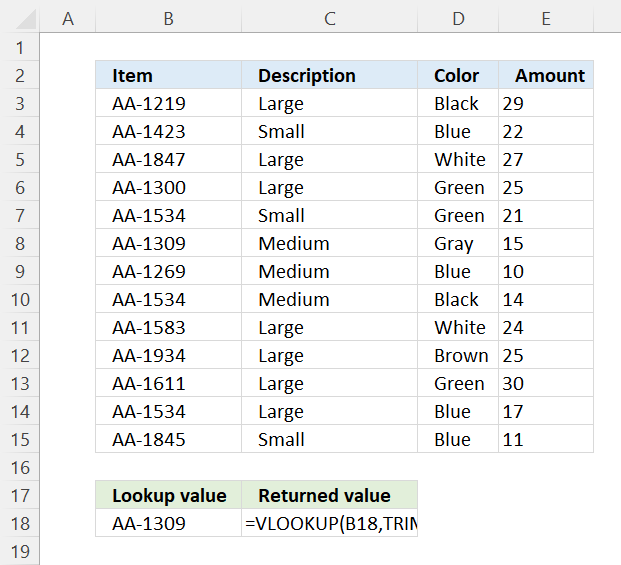There are several reasons why Excel might show the VLOOKUP formula in a cell instead of the result.

### 16.1 Cell formatted as text shows the formula

One common reason is that the cell is formatted as text, which means that Excel will display the formula as entered, rather than calculating the result.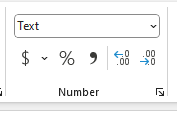To fix this go to tab "Home" on the ribbon. Press with left mouse button on the "Number Format" drop-down list and select "General".

### 16.2 "Show formula" is enabled by mistake

Another common reason this may happen is if you by mistake pressed CTRL + ` on your keyboard.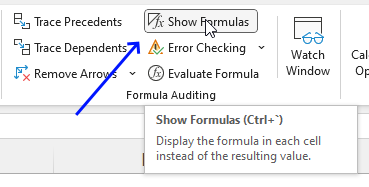You can undo it by pressing the same keys again or following these steps:

1. Go to tab "Formulas" on the ribbon.
2. Press with left mouse button on the "Show Formulas" button to disable this feature.

### 16.2 Formula contains a circular reference

Sometimes a circular reference may cause Excel to show the formula and not the result. A circular reference is when a formula refers back to its own cell, creating a loop that Excel cannot calculate.

1. Go to tab "Formulas" on the ribbon.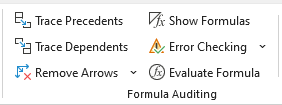2. Press with left mouse button on the "Trace Precedents" or "Trace Dependents" to find the reference causing this mess. Arrows show up on the worksheet assisting you to find the culprit.
3. Press with left mouse button on "Remove Arrows" button when the problem is solved.

## 17. VLOOKUP another sheet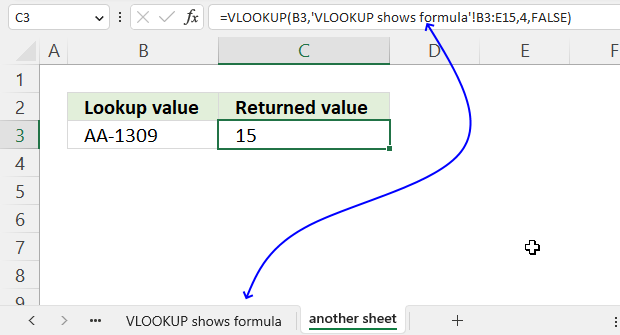The VLOOKUP function is not limited to one worksheet, you can reference data on another worksheet just as easily.

The VLOOKUP function has the following syntax:
VLOOKUP(lookup_value, table_array, col_index_num, [range_lookup])

The table_array argument references the data table you want to use, the example above demonstrates a reference to a worksheet named VLOOKUP shows formula.

There are certain rules you must follow in order to to create a reference:

1. The worksheet name must have a leading and trailing ' (apostrophe) if the name contains at least one space character. For example, 'VLOOKUP shows formula'
2. Have a reference to a cell or cell range.  For example, B3:E15
3. An exclamation mark ! between the worksheet name and the cell reference.

Example: 'VLOOKUP shows formula'!B3:E15

Here is the entire formula:

=VLOOKUP(B3,'VLOOKUP shows formula'!B3:E15,4,FALSE)

Tip! Use an absolute cell reference to keep it locked if you are going to copy the cell and paste it to the cells below. The \$ signs let you create an absolute cell reference, the formula becomes:

=VLOOKUP(B3,'VLOOKUP shows formula'!\$B\$3:\$E\$15,4,FALSE)

## 18. VLOOKUP - how to keep formatting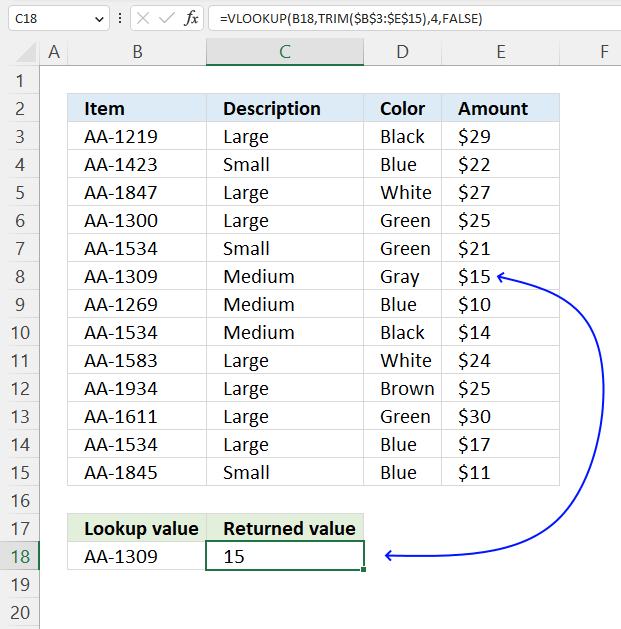The VLOOKUP formula can't keep the formatting from the source cell, this applies to all Excel functions for that matter.

You can, however, quickly copy the formatting and apply it to the formula cell. Here is how:

1. Select the source cell, in this example cell E8.
2. Go to tab "Home" on the ribbon.
3. Press with mouse on the "Format Painter" button.
4. Select the destination cell, in this example cell C18.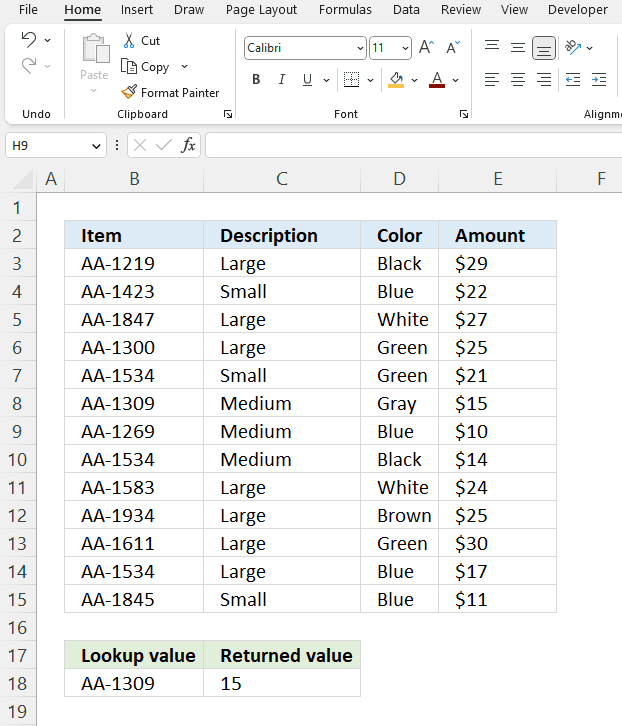## 19. VLOOKUP backward - how to perform a lookup from bottom to top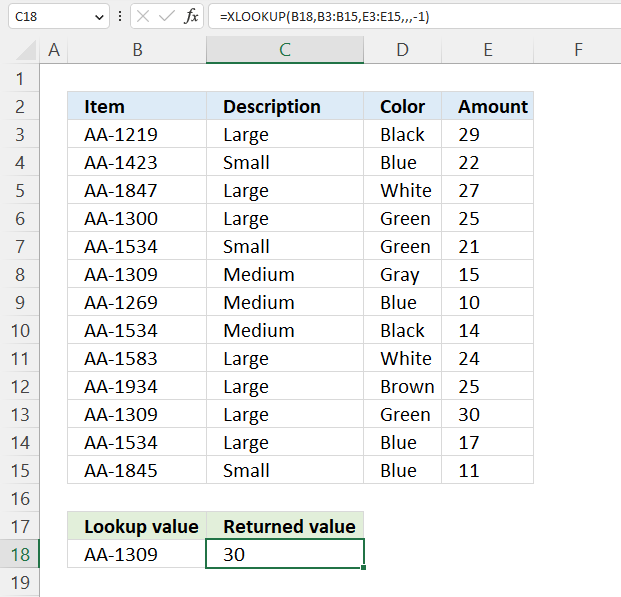The easiest way to do this is to use the XLOOKUP function.

Excel 365 dynamic array formula in cell C18:

=XLOOKUP(B18,B3:B15,E3:E15,,,-1)

The XLOOKUP function search one column for a given value, and return a corresponding value in another column from the same row.

Function syntax: XLOOKUP(lookup_value, lookup_array, return_array, [if_not_found], [match_mode], [search_mode])

If only VLOOKUP is available then I recommend flipping the data using a helper column. Here is how: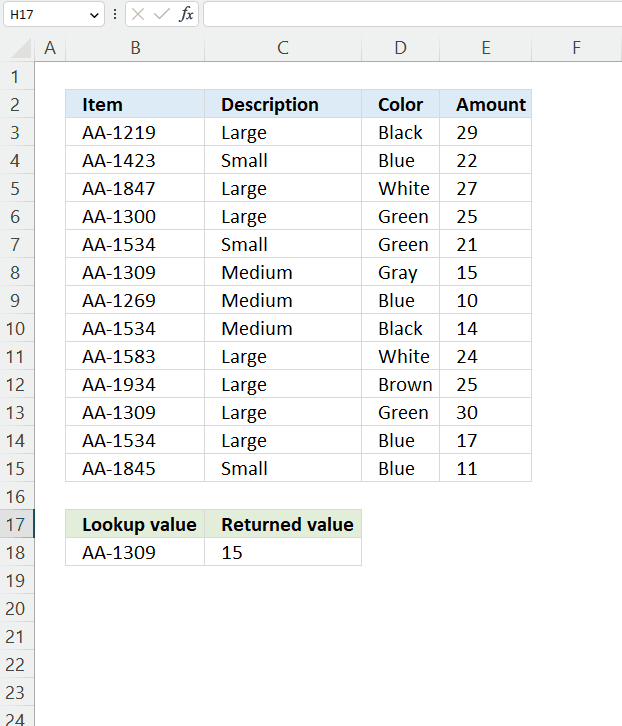1. Type 1 in a cell adjacent to the first row in the data set.
2. Go to the next cell below and type 2.
3. Select the cells containing 1 and 2.
4. Press and hold with left mouse button on the dot in the lower right corner of the selected cells.
5. Drag with mouse to the last row containing data.

You have now numbered each row from 1 to n.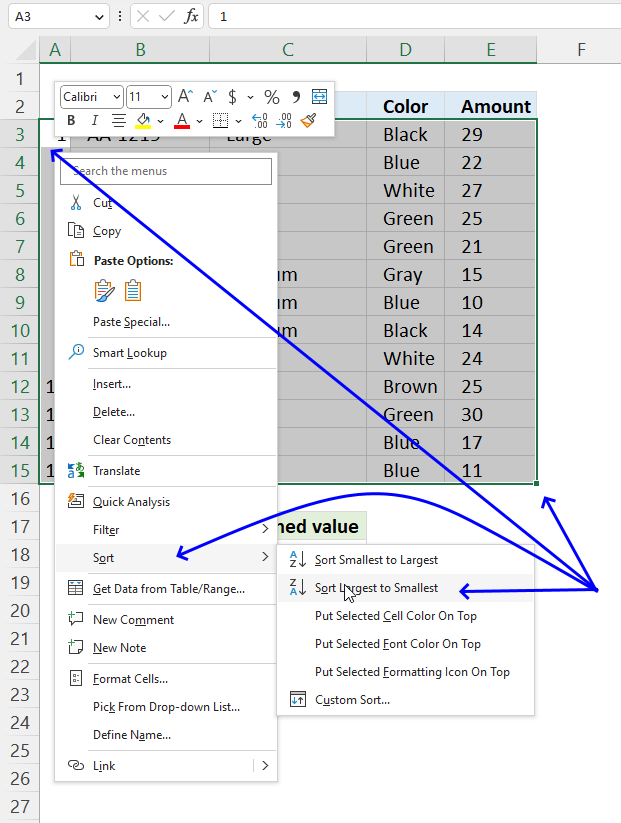It is now time to flip the entire data set.

1. Select the numbers and the data.
2. Press with right mouse button on with mouse on any of the numbers you created. A popup menu appears.
3. Press with left mouse button on "Sort", another popup menu appears.
4. Press with left mouse button on "Sort Largest to Smallest".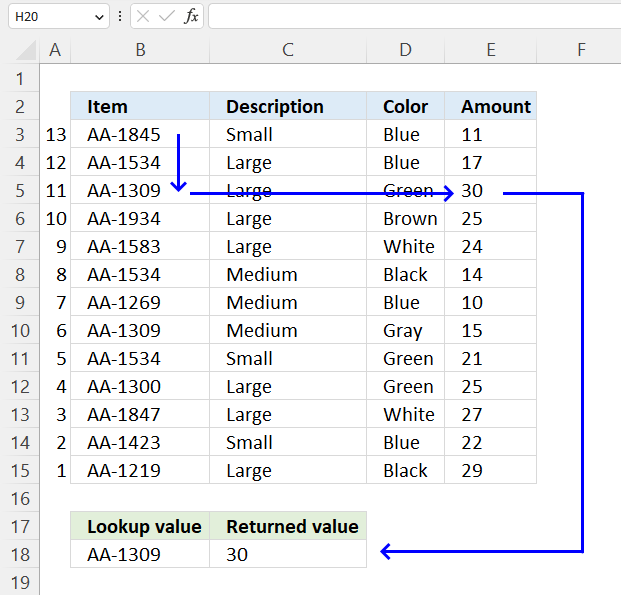## 20.VLOOKUP on two columns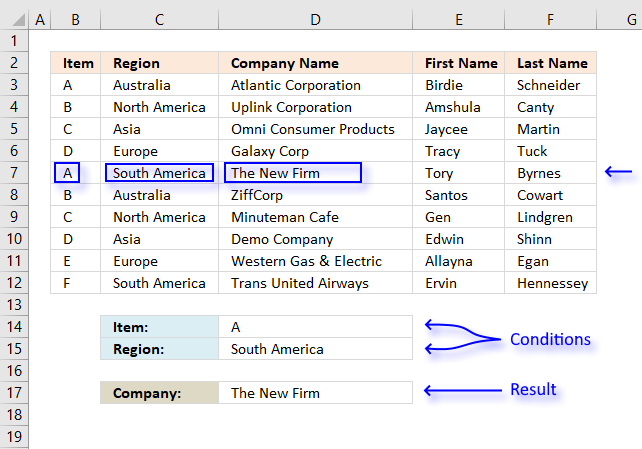Formula in cell D17:

=VLOOKUP(D14, IF(C3:C12=D15, B3:F12, ""), 3, FALSE)

I explain the formula in this post:
How to use VLOOKUP/XLOOKUP with multiple conditions

## 21. VLOOKUP between dates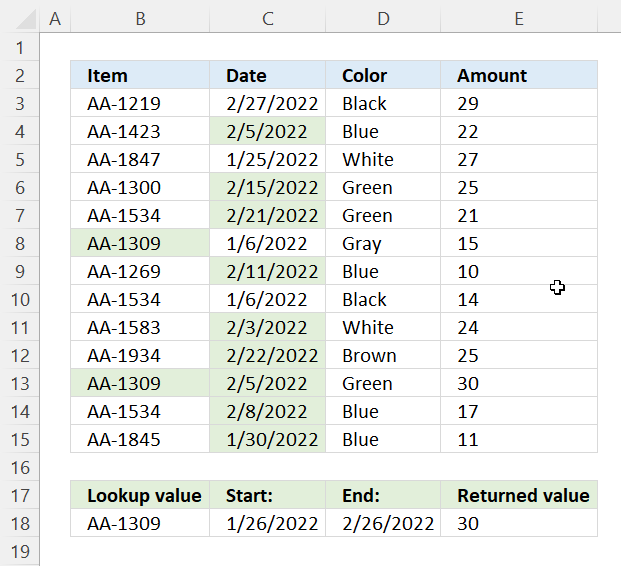The VLOOKUP formula in cell E3 performs a lookup on records that fall between the given start and end date. The lookup value is in cell B18, the date range is specified in cells C18:D18.

The conditionally formatted cells in columns B and C show which row matches all criteria.

Array formula in cell E18:

=VLOOKUP(B18, IF((C3:C15<=D18)*(C3:C15>=C18),\$B\$3:\$E\$15, ""), 4, FALSE)

### Explaining formula

#### Step 1 - First condition

The "less than" and equal sign check which dates in C3:C15 is smaller than or equal to the end date specified in cell D18. The result is an array of boolean values TRUE or FALSE.

C3:C15<=D18

becomes

{44619; 44597; 44586; 44607; 44613; 44567; 44603; 44567; 44595; 44614; 44597; 44600; 44591}<=44618

and returns

{FALSE; TRUE; TRUE; TRUE; TRUE; TRUE; TRUE; TRUE; TRUE; TRUE; TRUE; TRUE; TRUE}

#### Step 2 - Second condition

The "greater than" and equal sign combined check which dates in C3:C15 is greater than or equal to the start date specified in cell C18.

C3:C15>=C18

becomes

{44619; 44597; 44586; 44607; 44613; 44567; 44603; 44567; 44595; 44614; 44597; 44600; 44591}<=44618

and returns

{FALSE; TRUE; TRUE; TRUE; TRUE; TRUE; TRUE; TRUE; TRUE; TRUE; TRUE; TRUE; TRUE}

#### Step 3 - AND logic

The asterisk character multiplies numbers in an Excel formula, it also performs AND logic between boolean values and their equivalents.

(C3:C15<=D18)*(C3:C15>=C18)

becomes

{FALSE; TRUE; TRUE; TRUE; TRUE; TRUE; TRUE; TRUE; TRUE; TRUE; TRUE; TRUE; TRUE} * {FALSE; TRUE; TRUE; TRUE; TRUE; TRUE; TRUE; TRUE; TRUE; TRUE; TRUE; TRUE; TRUE}

and returns

{0; 1; 0; 1; 1; 0; 1; 0; 1; 1; 1; 1; 1}.

#### Step 4 - Evaluate IF function

The IF function returns one value if the logical test is TRUE and another value if the logical test is FALSE.

Function syntax: IF(logical_test, [value_if_true], [value_if_false])

IF((C3:C15<=D18)*(C3:C15>=C18),\$B\$3:\$E\$15,"")

becomes

IF({0; 1; 0; 1; 1; 0; 1; 0; 1; 1; 1; 1; 1},\$B\$3:\$E\$15,"")

and returns

{"AA-1219", 44619, "Black", 29; "AA-1423", 44597, "Blue", 22; "AA-1847", 44586, "White", 27; "AA-1300", 44607, "Green", 25; "AA-1534", 44613, "Green", 21; "AA-1309", 44567, "Gray", 15; "AA-1269", 44603, "Blue", 10; "AA-1534", 44567, "Black", 14; "AA-1583", 44595, "White", 24; "AA-1934", 44614, "Brown", 25; "AA-1309", 44597, "Green", 30; "AA-1534", 44600, "Blue", 17; "AA-1845", 44591, "Blue", 11}.

#### Step 5 - Evaluate VLOOKUP function

The VLOOKUP function lets you search the leftmost column for a value and return another value on the same row in a column you specify.

Function syntax: VLOOKUP(lookup_value, table_array, col_index_num, [range_lookup])

VLOOKUP(B18,IF((C3:C15<=D18)*(C3:C15>=C18),\$B\$3:\$E\$15,""),4,FALSE)

becomes

VLOOKUP("AA-1309",{"AA-1219", 44619, "Black", 29; "AA-1423", 44597, "Blue", 22; "AA-1847", 44586, "White", 27; "AA-1300", 44607, "Green", 25; "AA-1534", 44613, "Green", 21; "AA-1309", 44567, "Gray", 15; "AA-1269", 44603, "Blue", 10; "AA-1534", 44567, "Black", 14; "AA-1583", 44595, "White", 24; "AA-1934", 44614, "Brown", 25; "AA-1309", 44597, "Green", 30; "AA-1534", 44600, "Blue", 17; "AA-1845", 44591, "Blue", 11},4,FALSE)

and returns

30.

## 22. VLOOKUP returns #N/A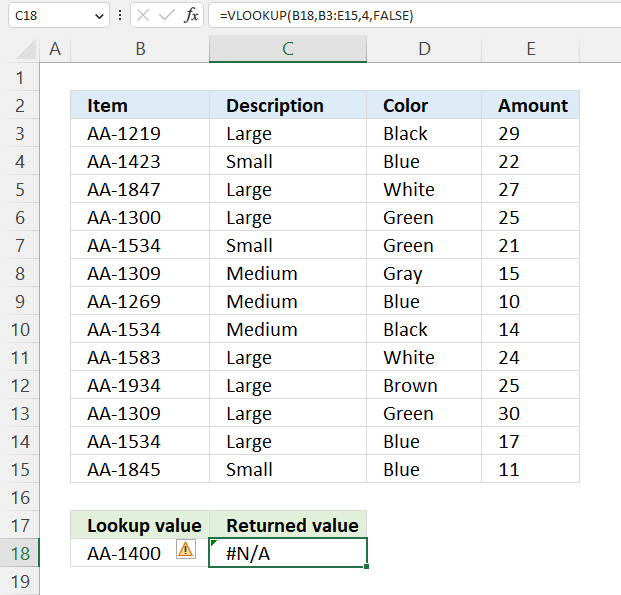VLOOKUP returns the #N/A error when the lookup value is not found in the leftmost column in the table_array. #N/A error means "value not available". This can happen if the lookup value is not in the first column of the range, the specified range is incorrect, or the lookup value contains extra spaces or is not entered exactly as it appears in the source data. It can also happen if the VLOOKUP formula is not entered correctly.

To fix this error, you can try the following:

• Make sure that the lookup value is in the first column of the specified range.
• Make sure the lookup value is entered exactly as it appears in the source data, with no extra spaces. Tip! Use the TRIM function to remove possible extra spaces, if needed.
• Check the syntax of the VLOOKUP formula to ensure it is correct and all the arguments are in the correct order.

Formula in cell C18:

=VLOOKUP(B18,B3:E15,4,FALSE)

The above example demonstrates a lookup value that doesn't exist in the leftmost column in cell range B3:E15.

## 23. VLOOKUP yes if true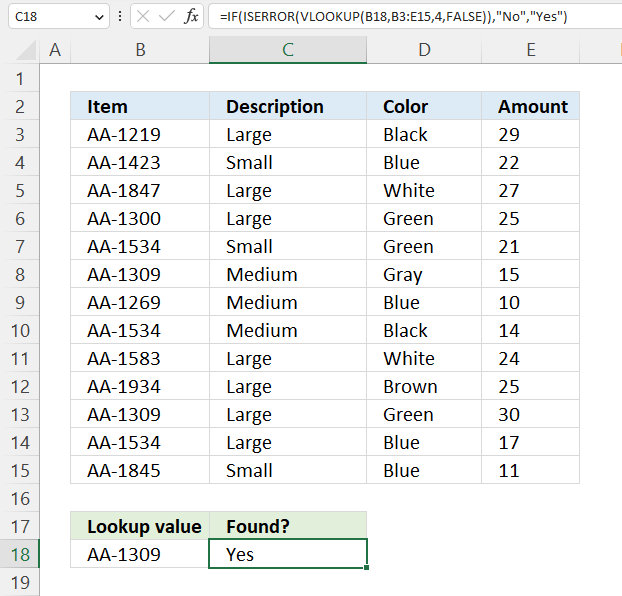The formula in cell C18 uses the IF, ISERROR, and VLOOKUP functions to check if a lookup value is found in a specified range, and return Yes if true and no if false.

Formula in cell C18:

=IF(ISERROR(VLOOKUP(B18,B3:E15,4,FALSE)),"No","Yes")

This formula is using VLOOKUP to search for a value in a range, and then using IF and ISERROR to return custom values depending on whether the lookup value is found or not.

### Explaining formula

#### Step 1 - Evaluate VLOOKUP function

The VLOOKUP function lets you search the leftmost column for a value and return another value on the same row in a column you specify.

Function syntax: VLOOKUP(lookup_value, table_array, col_index_num, [range_lookup])

The VLOOKUP function is used to search for the value in cell B18 in the range B3:E15. It is looking for an exact match, so the range_lookup argument is set to FALSE.  The formula is looking for a match in the fourth column of the range, so the col_index_num argument is set to 4.

If the lookup value is found, VLOOKUP will return the corresponding value from the fourth column of the range. If the lookup value is not found, VLOOKUP will return the #N/A error.

VLOOKUP(B18,B3:E15,4,FALSE))

returns 15.

"AA-1309" is found in cell B13, the corresponding cell on the same row in column 4 in cell range B3:E15 is 15.

#### Step 2 - Check if VLOOKUP returns an error

The ISERROR function returns TRUE if a cell contains an error.

Function syntax: ISERROR(value)

ISERROR(VLOOKUP(B18,B3:E15,4,FALSE))

becomes

ISERROR(15)

and returns FALSE.

If VLOOKUP returns the #N/A error, ISERROR will return TRUE. Otherwise, it will return FALSE.

#### Step 3 - Check if value is TRUE or FALSE

The IF function returns one value if the logical test is TRUE and another value if the logical test is FALSE.

Function syntax: IF(logical_test, [value_if_true], [value_if_false])

IF(ISERROR(VLOOKUP(B18,B3:E15,4,FALSE)),"No","Yes")

becomes

IF(FALSE,"No","Yes")

and returns "Yes".

The IF function is then used to check the result of the ISERROR function. If ISERROR returns TRUE, the IF function will return the text "No". Otherwise, it will return the text "Yes".

## 24. VLOOKUP part of text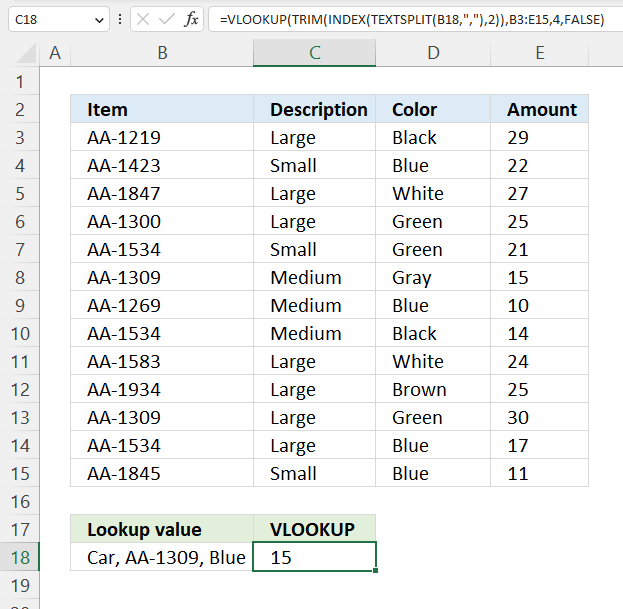This formula is using the TEXTSPLIT and INDEX functions to extract a substring from cell B18, then uses TRIM and VLOOKUP to search for that substring in a range and return the corresponding value from a different column.

Excel 365 formula in cell C18:

=VLOOKUP(TRIM(INDEX(TEXTSPLIT(B18,","),2)),B3:E15,4,FALSE)

### Explaining formula

#### Step 1 - Split value in cell B18 based on a delimiting value

The TEXTSPLIT function splits a string into an array based on delimiting values.

Function syntax: TEXTSPLIT(Input_Text, col_delimiter, [row_delimiter], [Ignore_Empty])

TEXTSPLIT(B18,",")

The TEXTSPLIT function is used to split the text in cell B18 at each comma and return an array of substrings.

#### Step 2 - Get second value in the array

The INDEX function returns a value or reference from a cell range or array, you specify which value based on a row and column number.

Function syntax: INDEX(array, [row_num], [column_num])

INDEX(TEXTSPLIT(B18,","),2)

The INDEX function is used to extract the second element of the array returned by TEXTSPLIT, which is the substring after the first comma.

#### Step 3 - Remove leading and trailing spaces

The TRIM function deletes all blanks or space characters except single blanks between words in a cell value.

Function syntax: TRIM(text)

TRIM(INDEX(TEXTSPLIT(B18,","),2))

The TRIM function is then used to remove any leading or trailing spaces from the substring.

#### Step 4 - Evaluate VLOOKUP function

The VLOOKUP function lets you search the leftmost column for a value and return another value on the same row in a column you specify.

Function syntax: VLOOKUP(lookup_value, table_array, col_index_num, [range_lookup])

VLOOKUP(TRIM(INDEX(TEXTSPLIT(B18,","),2)),B3:E15,4,FALSE)

The VLOOKUP function is then used to search for the trimmed substring in the range B3:E15. It is looking for an exact match, so the range_lookup argument is set to FALSE. The formula is looking for a match in the fourth column of the range, so the col_index_num argument is set to 4.

If the lookup value is found, VLOOKUP will return the corresponding value from the fourth column of the range.

## 25. VLOOKUP vs INDEX MATCH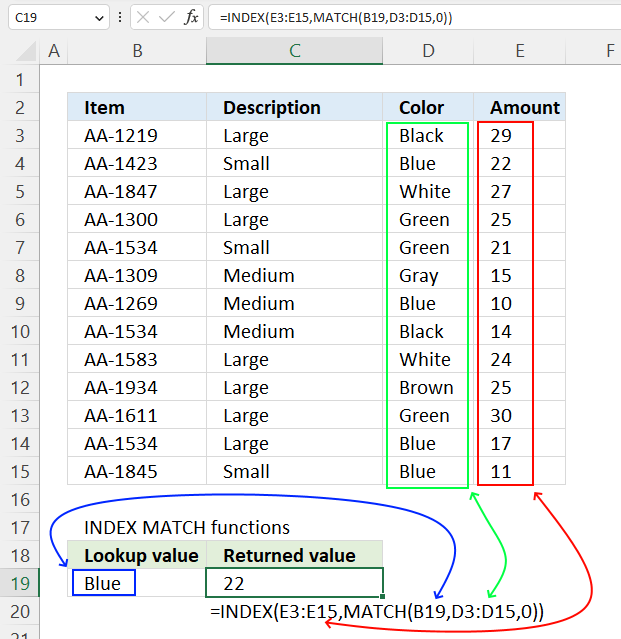VLOOKUP and INDEX MATCH are both functions in Excel that can be used to find information in a table or range of cells. VLOOKUP stands for "vertical lookup", and it is used to find information in any column based on a lookup value that is located in the leftmost column of a given cell range. It requires a lookup value, a range of cells that contains the lookup value, and a number determining what column contains the information to be returned.

INDEX MATCH also allows you to look up values in a table based on a lookup value. It is more flexible than VLOOKUP because it allows you to search for values in any column, not just the first column of the table.

The INDEX MATCH is considered to be a more powerful and flexible alternative to VLOOKUP because it can handle more complex lookup scenarios using a condition or criteria. However, VLOOKUP is often faster and easier to use, so it is a good choice for simple lookup tasks as long as you know its limitations.

Formula in cell C19:

=INDEX(E3:E15,MATCH(B19,D3:D15,0))

### Explaining formula

The INDEX function returns the value in a given cell in a range of cells, while the MATCH function returns the position of a value in a given range of cells.

In this case, the formula is returning the value in the range E3:E15 that corresponds to the position of the value in B19 in the range D3:D15.

The 0 (zero) argument in the MATCH function specifies that the function should perform an exact match.

#### Step 1 - Find the position of a given value

The MATCH function returns the relative position of an item in an array that matches a specified value in a specific order.

Function syntax: MATCH(lookup_value, lookup_array, [match_type])

MATCH(B19,D3:D15,0)

becomes

MATCH("Blue",{"Black";"Blue";"White";"Green";"Green";"Gray";"Blue";"Black";"White";"Brown";"Green";"Blue";"Blue"},0)

and returns 2.

#### Step 2 - Get value based on the position

The INDEX function returns a value or reference from a cell range or array, you specify which value based on a row and column number.

Function syntax: INDEX(array, [row_num], [column_num])

INDEX(E3:E15,MATCH(B19,D3:D15,0))

becomes

INDEX({29; 22; 27; 25; 21; 15; 10; 14; 24; 25; 30; 17; 11},2)

and returns 22.

## 26. VLOOKUP returns multiple columns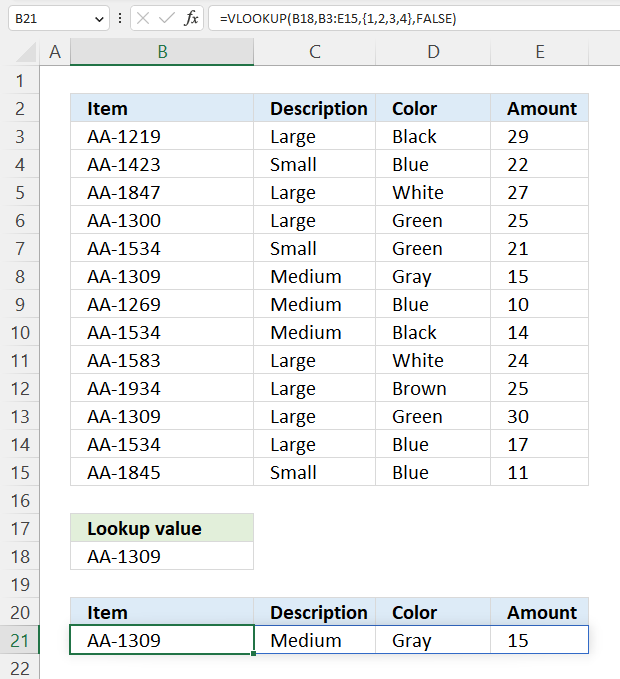To use VLOOKUP and return values from multiple columns, you can create an array containing the corresponding column numbers you want to extract data from.

The third argument lets you specify which column to use, however, it is also possible to specify multiple columns.

Formula in cell B21:

=VLOOKUP(B18, B3:E15, {1, 2, 3, 4}, FALSE)

This formula is an array formula, you need to enter it like this:

1. Select cell range B21:E21.
2. Type the above formula.

To enter an array formula, you need to press Ctrl+Shift+Enter instead of just Enter after typing the formula. This tells Excel that the formula is an array formula, and it will automatically enclose the formula in braces ({}) to indicate that it is an array formula.

Excel 365 users can enter the formula as a regular formula, press Enter only. The formula expands automatically to the right, Microsoft calls this spilling.

### Explaining the formula

#### Step 1 - Create an array

The curly brackets let you create a hardcoded array in a formula. Text values must be enclosed with double quotes ", however, we are using numbers in this case.

The comma is a delimiting character that separates values into columns. The corresponding character for rows is a semi-colon ;.  These characters is determined by your regional settings and therefore may be different.

{1, 2, 3, 4}

#### Step 2 - Evaluate the VLOOKUP function

The VLOOKUP function lets you search the leftmost column for a value and return another value on the same row in a column you specify.

Function syntax: VLOOKUP(lookup_value, table_array, col_index_num, [range_lookup])

VLOOKUP(B18, B3:E15, {1, 2, 3, 4}, FALSE)

returns

{"AA-1309", "Medium", "Gray", 15}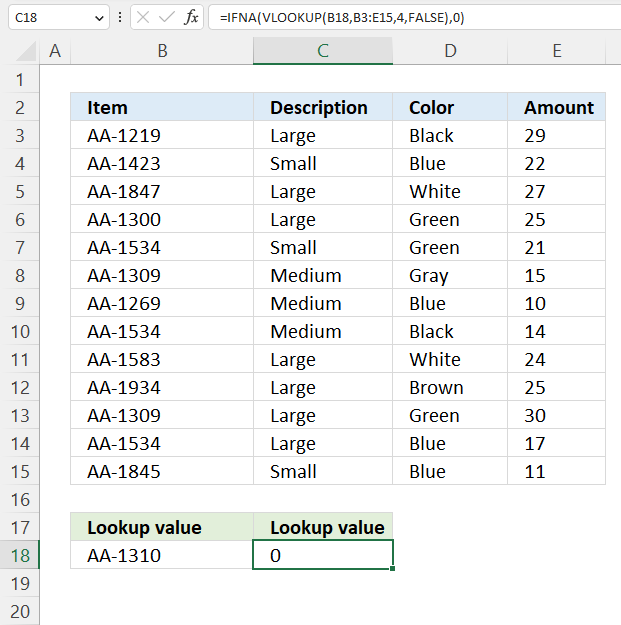To return a value of 0 (zero) if the VLOOKUP function is unable to find the lookup value, you can use the IFNA function in combination with VLOOKUP. The IFNA function will check the result of the VLOOKUP function, and if it is an error it will return a value of 0 (zero) instead.

Formula in cell B18:

=IFNA(VLOOKUP(B18,B3:E15,4,FALSE),0)

The image above demonstrates a lookup value that doesn't exist in the lookup column, the formula returns 0 (zero) instead of a #N/A error.

### Explaining formula

#### Step 1 - Evaluate the VLOOKUP function

The VLOOKUP function lets you search the leftmost column for a value and return another value on the same row in a column you specify.

Function syntax: VLOOKUP(lookup_value, table_array, col_index_num, [range_lookup])

VLOOKUP(B18,B3:E15,4,FALSE)

returns #N/A

#### Step 2 - Check if the result is a #N/A error

The IFNA function handles #N/A errors only, it returns a specific value if the formula returns a #N/A error.

Function syntax: IFNA(value, value_if_na)

IFNA(VLOOKUP(B18,B3:E15,4,FALSE),0)

becomes

IFNA(#N/A,0)

and returns 0 (zero).

## 28. VLOOKUP sum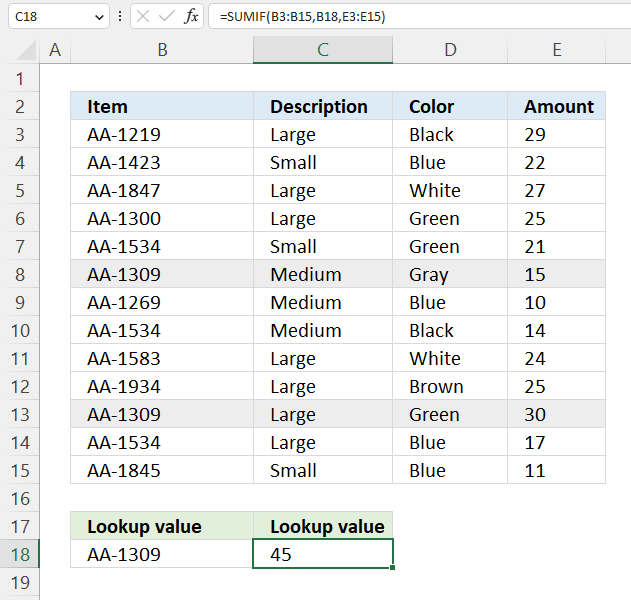The VLOOKUP function is not designed to be used for adding numbers. VLOOKUP is a lookup and reference function that is used to lookup a value in a cell range and return a corresponding value from another column in the same row. It does not have any built-in functionality for calculating totals, however, Excel has functions that are built for this, and they are the SUMIF and SUMIFS functions.

Formula in cell B18:

=SUMIF(B3:B15,B18,E3:E15)

The SUMIF function sums numerical values based on a condition.

Function syntax: SUMIF(range, criteria, [sum_range])

The SUMIF function is a function that adds up the values in a range of cells that meet certain criteria. It is similar to the SUM function, but it allows you to specify a condition that must be met in order for a value to be included in the sum.

To use the SUMIF function, you need to specify the following three arguments:

1. The range of cells to sum.
2. The criteria to use for determining which cells to include in the sum.
3. An optional range of cells to sum.

## 29. VLOOKUP true false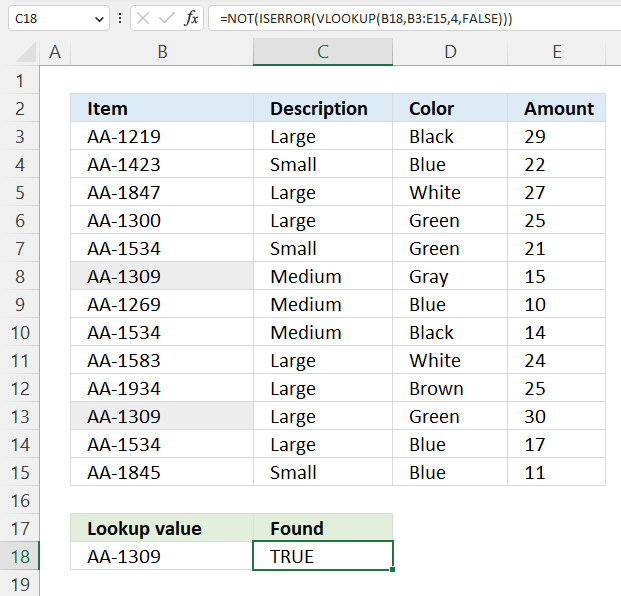This example shows a formula that returns TRUE if a value is found and FALSE if not found using the VLOOKUP function.

Formula in cell C18:

=NOT(ISERROR(VLOOKUP(B18,B3:E15,4,FALSE)))

The above formula is overly complicated for this simple task, here is smaller formula:

=OR(B18=B3:B15)

### Explaining the VLOOKUP formula

#### Step 1 - Perform a VLOOKUP

The VLOOKUP function lets you search the leftmost column for a value and return another value on the same row in a column you specify.

Function syntax: VLOOKUP(lookup_value, table_array, col_index_num, [range_lookup])

VLOOKUP(B18,B3:E15,4,FALSE)

returns 15.

#### Step 2 - Check if it returns an error

The ISERROR function returns TRUE if a cell contains an error.

Function syntax: ISERROR(value)

ISERROR(VLOOKUP(B18,B3:E15,4,FALSE))

becomes

ISERROR(15)

and returns FALSE.

#### Step 3 - Change boolean value from TRUE to FALSE or vice versa

The NOT function returns the boolean opposite to the given argument.

Function syntax: NOT(logical)

NOT(ISERROR(VLOOKUP(B18,B3:E15,4,FALSE)))

becomes

NOT(FALSE)

and returns TRUE.

## 30. VLOOKUP unique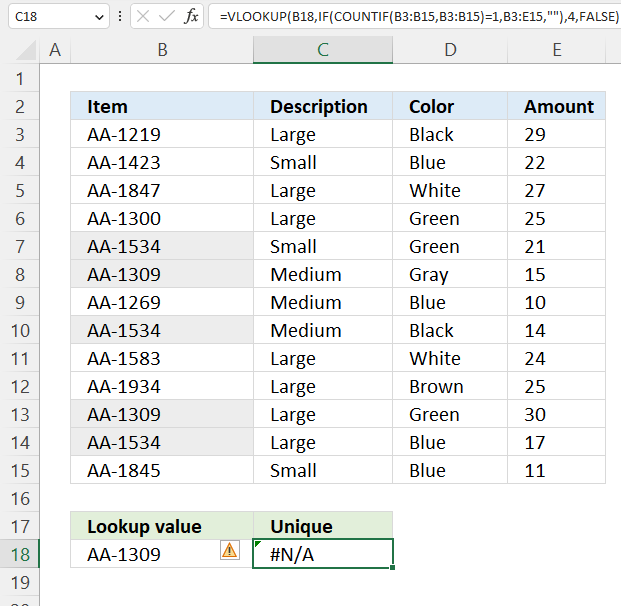This formula in cell C18 filters unique values in cell range B3:B15 meaning the value only exists once. Then performs a VLOOKUP and returns the corresponding value in cells E3:E15.

Array formula in cell C18:

=VLOOKUP(B18,IF(COUNTIF(B3:B15,B3:B15)=1,B3:E15,""),4,FALSE)

This formula returns a #N/A error because lookup value "AA-1309" specified in cell B18 has a duplicate. The value can be found in both cells B8 and B13, this makes the formula filter out these records and the VLOOKUP function cant find the lookup value returning an error.

Check section 26 on how to enter an array formula, Excel 365 users can enter this formula as a regular formula.

### Explaining formula

#### Step 1 - Count cells based on the same cells

The COUNTIF function calculates the number of cells that is equal to a condition.

Function syntax: COUNTIF(range, criteria)

COUNTIF(B3:B15,B3:B15)

becomes

COUNTIF({"AA-1219"; "AA-1423"; "AA-1847"; "AA-1300"; "AA-1534"; "AA-1309"; "AA-1269"; "AA-1534"; "AA-1583"; "AA-1934"; "AA-1309"; "AA-1534"; "AA-1845"},{"AA-1219"; "AA-1423"; "AA-1847"; "AA-1300"; "AA-1534"; "AA-1309"; "AA-1269"; "AA-1534"; "AA-1583"; "AA-1934"; "AA-1309"; "AA-1534"; "AA-1845"})

and returns the following array:

{1; 1; 1; 1; 3; 2; 1; 3; 1; 1; 2; 3; 1}

#### Step 2 - Check if value is unique

The equal sign allows you to compare value to value, it also works with an array and a value. The result is an array containing boolean value TRUE or FALSE.

COUNTIF(B3:B15,B3:B15)=1

becomes

{1; 1; 1; 1; 3; 2; 1; 3; 1; 1; 2; 3; 1}=1

and returns

{TRUE; TRUE; TRUE; TRUE; FALSE; FALSE; TRUE; FALSE; TRUE; TRUE; FALSE; FALSE; TRUE}.

#### Step 3 - Filter out duplicate values

The IF function returns one value if the logical test is TRUE and another value if the logical test is FALSE.

Function syntax: IF(logical_test, [value_if_true], [value_if_false])

IF(COUNTIF(B3:B15,B3:B15)=1,B3:E15,"")

becomes

IF({TRUE; TRUE; TRUE; TRUE; FALSE; FALSE; TRUE; FALSE; TRUE; TRUE; FALSE; FALSE; TRUE},{"AA-1219","Large","Black",29; "AA-1423","Small","Blue",22; "AA-1847","Large","White",27; "AA-1300","Large","Green",25; "AA-1534","Small","Green",21; "AA-1309","Medium","Gray",15; "AA-1269","Medium","Blue",10; "AA-1534","Medium","Black",14; "AA-1583","Large","White",24; "AA-1934","Large","Brown",25; "AA-1309","Large","Green",30; "AA-1534","Large","Blue",17; "AA-1845","Small","Blue",11},"")

and returns

{"AA-1219","Large","Black",29; "AA-1423","Small","Blue",22; "AA-1847","Large","White",27; "AA-1300","Large","Green",25; "","","",""; "","","",""; "AA-1269","Medium","Blue",10; "","","",""; "AA-1583","Large","White",24; "AA-1934","Large","Brown",25; "","","",""; "","","",""; "AA-1845","Small","Blue",11}

#### Step 4 - Perform a VLOOKUP

The VLOOKUP function lets you search the leftmost column for a value and return another value on the same row in a column you specify.

Function syntax: VLOOKUP(lookup_value, table_array, col_index_num, [range_lookup])

VLOOKUP(B18,IF(COUNTIF(B3:B15,B3:B15)=1,B3:E15,""),4,FALSE)

becomes

VLOOKUP("AA-1309", {"AA-1219","Large","Black",29; "AA-1423","Small","Blue",22; "AA-1847","Large","White",27; "AA-1300","Large","Green",25; "","","",""; "","","",""; "AA-1269","Medium","Blue",10; "","","",""; "AA-1583","Large","White",24; "AA-1934","Large","Brown",25; "","","",""; "","","",""; "AA-1845","Small","Blue",11}, 4, FALSE)

returns #N/A!

## Get Excel *.xlsm file

VLOOKUP function.xlsm